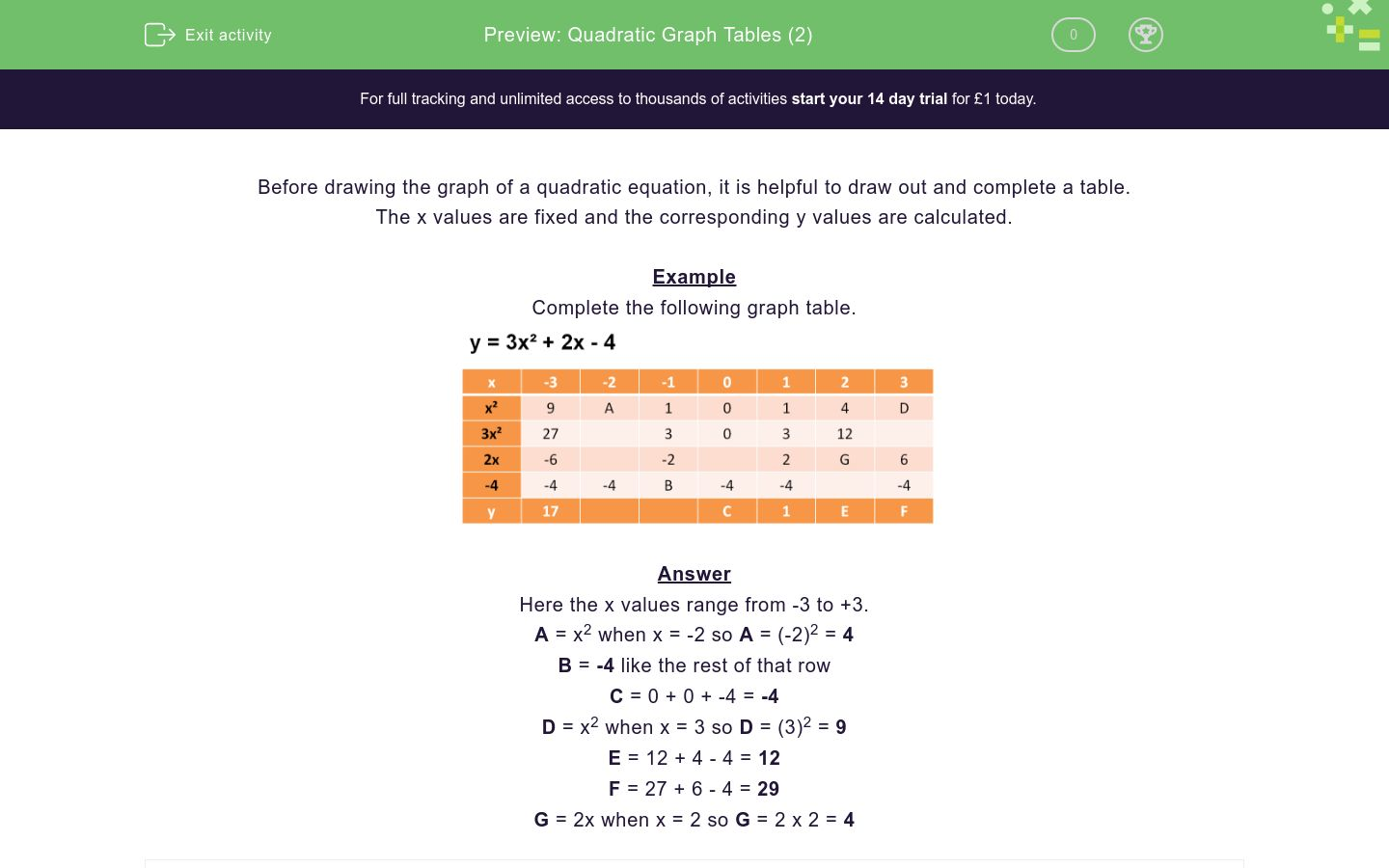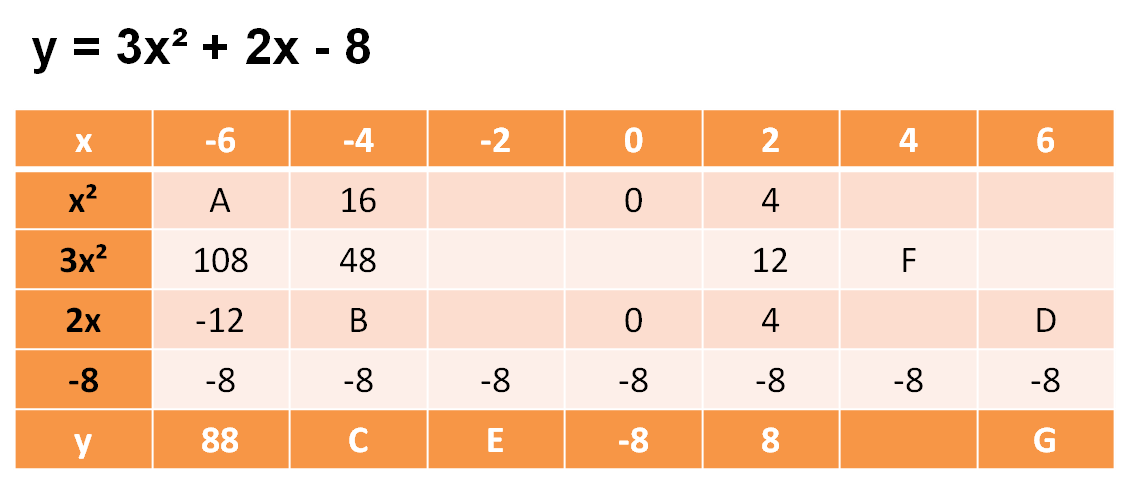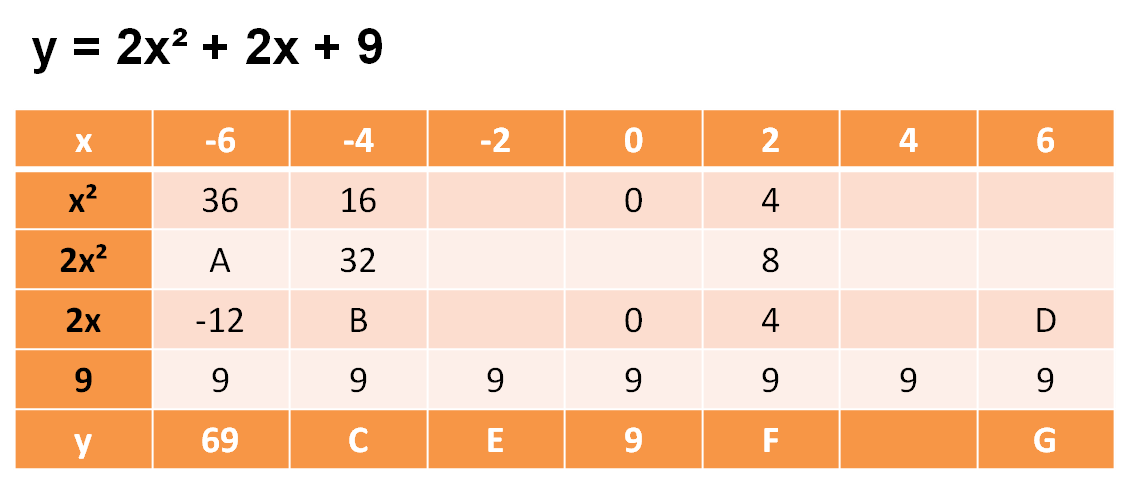### Affordable pricing from £10/month

In this worksheet, students complete tables for quadratic graphs.Key stage:  KS 4

Curriculum topic:  Algebra

Difficulty level:### QUESTION 1 of 10

Before drawing the graph of a quadratic equation, it is helpful to draw out and complete a table.

The x values are fixed and the corresponding y values are calculated.

Example

Complete the following graph table.Here the x values range from -3 to +3.

A = x2 when x = -2 so A = (-2)2 = 4

B = -4 like the rest of that row

C = 0 + 0 + -4 = -4

D = x2 when x = 3 so D = (3)2 = 9

E = 12 + 4 - 4 = 12

F = 27 + 6 - 4 = 29

G = 2x when x = 2 so G = 2 x 2 = 4

Complete the following graph table by matching the correct values to the letters.## Column B

A
9
B
4
C
-4
D
11
E
4
F
-4
G
4

Complete the following graph table by matching the correct values to the letters.## Column B

A
-1
B
4
C
-4
D
9
E
-4
F
-4
G
-4

Complete the following graph table by matching the correct values to the letters.## Column B

A
4
B
9
C
4
D
9
E
-6
F
8
G
2

Complete the following graph table by matching the correct values to the letters.## Column B

A
-9
B
12
C
-4
D
9
E
12
F
9
G
23

Complete the following graph table by matching the correct values to the letters.## Column B

A
1
B
-4
C
4
D
E
9
F
1
G
-3½

Complete the following graph table by matching the correct values to the letters.## Column B

A
12
B
0
C
112
D
48
E
36
F
-8
G
32

Complete the following graph table by matching the correct values to the letters.## Column B

A
36
B
88
C
48
D
8
E
-12
F
0
G
8

Complete the following graph table by matching the correct values to the letters.## Column B

A
12
B
93
C
-8
D
21
E
72
F
13
G
33

Complete the following graph table by matching the correct values to the letters.## Column B

A
4
B
72
C
70
D
2
E
3
F
25
G
-2

Complete the following graph table by matching the correct values to the letters.## Column B

A
27
B
11
C
1
D
21
E
27
F
-1
G
2
• Question 1

Complete the following graph table by matching the correct values to the letters.## Column B

A
4
B
-4
C
-4
D
4
E
9
F
11
G
4
• Question 2

Complete the following graph table by matching the correct values to the letters.## Column B

A
4
B
-4
C
-4
D
-4
E
9
F
-1
G
-4
• Question 3

Complete the following graph table by matching the correct values to the letters.## Column B

A
9
B
4
C
8
D
-6
E
9
F
4
G
2
• Question 4

Complete the following graph table by matching the correct values to the letters.## Column B

A
9
B
-4
C
-9
D
12
E
9
F
23
G
12
• Question 5

Complete the following graph table by matching the correct values to the letters.## Column B

A
9
B
-4
C
-3½
D
1
E
4
F
G
1
• Question 6

Complete the following graph table by matching the correct values to the letters.## Column B

A
36
B
-8
C
32
D
12
E
0
F
48
G
112
• Question 7

Complete the following graph table by matching the correct values to the letters.## Column B

A
36
B
8
C
48
D
-12
E
8
F
0
G
88
• Question 8

Complete the following graph table by matching the correct values to the letters.## Column B

A
72
B
-8
C
33
D
12
E
13
F
21
G
93
• Question 9

Complete the following graph table by matching the correct values to the letters.## Column B

A
72
B
-2
C
25
D
3
E
2
F
4
G
70
• Question 10

Complete the following graph table by matching the correct values to the letters.## Column B

A
27
B
2
C
11
D
27
E
1
F
-1
G
21
---- OR ----

Sign up for a £1 trial so you can track and measure your child's progress on this activity.

### What is EdPlace?

We're your National Curriculum aligned online education content provider helping each child succeed in English, maths and science from year 1 to GCSE. With an EdPlace account you’ll be able to track and measure progress, helping each child achieve their best. We build confidence and attainment by personalising each child’s learning at a level that suits them.